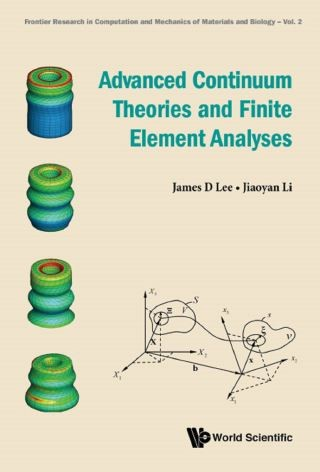## Advanced Continuum Theories and Finite Element Analyses• Description
• Author
• Info
• Reviews

### Description

This comprehensive volume presents a unified framework of continuum theories. It indicates that (i) microcontinuum theories (micromorphic and micropolar theories) are natural extension of classical continuum mechanics, and (ii) classical continuum mechanics is a special case of microcontinuum theories when the deformable material point is idealized as a single mathematical point. The kinematics and basic laws are rigorously derived. Based on axiomatic approach, constitutive theory is systematically derived for various kinds of materials, ranging from Stokesian fluid to thermo-visco-elastic-plastic solid. Material force and Thermomechanical-electromagnetic coupling are introduced and discussed. Moreover, general finite element methods for large-strain thermomechanical coupling physical phenomena are systematically formulated. Also, non-classical continuum theories (Nonlocal Theory, Mechanobiology, 4D printing, Poromechanics, and Non-Self-Similar Crack Propagation) are rigorously formulated with applications and demonstrated numerically.

As an advanced monograph, this unique compendium can also be used as a textbook for several graduate courses, including continuum mechanics, finite element methods, and advanced engineering science theories. Extensive problems are provided to help students to better understand the topics covered.

Contents:
• Classical Continuum Mechanics:
• Kinematics of Classical Continuum Mechanics
• Stresses
• Balance Laws
• Constitutive Theory
• Simple Thermoviscoelasticity
• Fluid Dynamics
• Thermoelasticity and Viscoelasticity
• Elasticity
• Plasticity
• Electromagnetic Interactions
• Microcontinuum Field Theories:
• Kinematics of Microcontinuum Field Theories
• Stresses and Balance Laws
• Constitutive Theory
• Thermomechanical Materials
• Micromorphic and Micropolar Fluids
• Micromorphic Plasticity
• Material Force
• Thermomechanical-Electromagnetic Coupling
• Finite Element Analyses:
• Preliminaries
• Finite Element Formulation of Classical Continuum Mechanics
• Dynamic Crack Propagation
• Finite Element Formulation of General Micromorphic Theory
• Finite Element Formulation of Linear Micromorphic Theory
• Passage to Finite Element Formulation of Micropolar Theory
• Special Topics:
• Nonlocal Theory
• Mechanobiology
• 4D Printing
• Poroelasticity
• Nematic Liquid Crystal
• Appendices:
• Tensor Analysis
• Representation Theorem

Readership: Professionals, researchers, academics, and graduate students in engineering mechanics, mechanical engineering, general materials science, continuum physics and mathematical modeling.Continuum Mechanics;Microcontinuum Theories;Finite Element Analyses;Mechanobiology;Nonlocal Theory;Poromechanics00